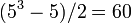# PSL(2,5) is isomorphic to A5

This article gives a proof/explanation of the equivalence of multiple definitions for the term alternating group:A5
View a complete list of pages giving proofs of equivalence of definitions

## Statement

The projective special linear group of degree two over field:F5, which we denote$PSL(2,5)$, is isomorphic to alternating group:A5.

## Facts used

1.$A_5$ has order$5!/2 = 60$
2.$PSL(2,5)$ has order$(5^3 - 5)/2 = 60$ by order formulas for linear groups of degree two
3. A5 is the unique simple non-abelian group of smallest order: This says that$A_5$ is the simple non-abelian group of smallest possible order and also that any simple non-abelian group of the same order as$A_5$ must be isomorphic to$A_5$.
4. Projective special linear groups are simple with some exceptions, but$PSL(2,5)$ is not among the exceptions, so$PSL(2,5)$ is simple.

## Proof

The proof follows directly by combining Facts (1)-(4).